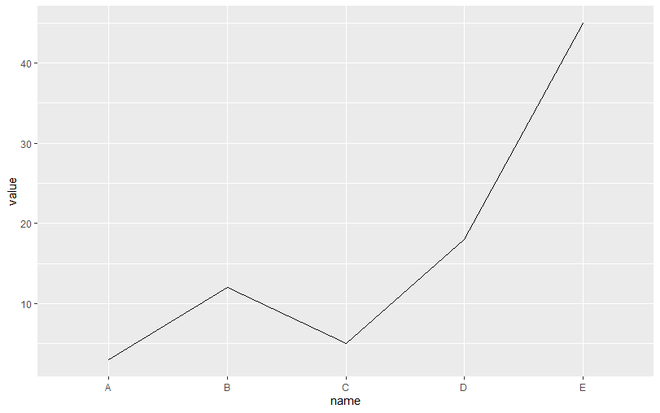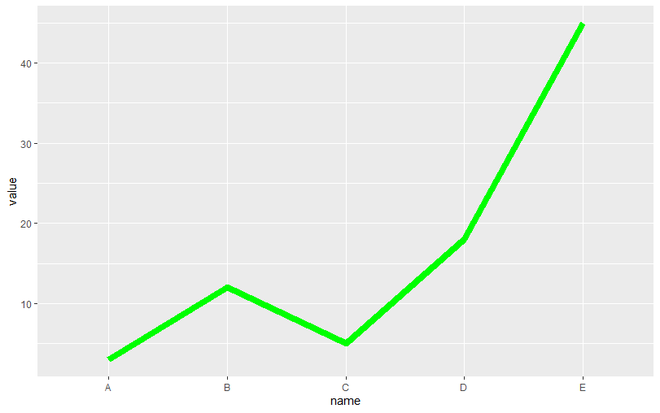# How to change line width in ggplot2?

• Last Updated : 03 Mar, 2021

ggplot2 is a data visualization package for the statistical programming language R. This article discusses how can we change line width in ggplot2. For this, only the size parameter in the geom_line() function has to be initialized to the required value.

Syntax:

geom_line(mapping=NULL, data=NULL, stat=”identity”, position=”identity”,…)

Approach

• Import module
• Create data frame
• Create plot with a line
• Display plot

Let us first draw a regular plot so that the difference is better understandable and apparent.

Example:

## R

 `library``(ggplot2)`` ` `data <- ``data.frame``(``  ``name=``c``(``"A"``,``"B"``,``"C"``,``"D"``,``"E"``),  ``  ``value=``c``(3,12,5,18,45)``)`` ` `# For Create a simple line graph``ggplot``(data, ``aes``(x=name, y=value, group=1)) + ``  ``geom_line``()`

Output:Simple line graph using ggplot2

Setting the required value to the size parameter of geom_line() will produce a line plot of the required width.

Example:

## R

 `library``(ggplot2)`` ` `data <- ``data.frame``(``  ``name=``c``(``"A"``,``"B"``,``"C"``,``"D"``,``"E"``),  ``  ``value=``c``(3,12,5,18,45)``)`` ` `# For create line graph with change of size and color.``ggplot``(data, ``aes``(x=name, y=value, group=1)) + ``  ``geom_line``(size=3, color=``"green"``)`

Output:Modified Line Graph using ggplot2

My Personal Notes arrow_drop_up